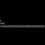# Stamp and Truck Question

1) An envelope has space for at most three stamps. If you are given three stamps of denomination 1, and three stamps of denomination a(a>1) the least positive integer for which there is no stamp value is........

2) Ten trucks numbered 1 to 10, are carrying packets of sugar. Each packet weight either 999g or 1000g and each truck carries only packets of equal weights. The combined weight of 1packet selected from the first truck,2 packets selected from the second, 4 packet from the third, and so on and 2^9 packets from the tenth truck is 1022870g. The truck that have the lighter bags are: (A)1,3,5 (B)2,4,5 (C)1,9 (D)2,8

How to do this problem??Note by Rajath Krishna R
7 years ago

This discussion board is a place to discuss our Daily Challenges and the math and science related to those challenges. Explanations are more than just a solution — they should explain the steps and thinking strategies that you used to obtain the solution. Comments should further the discussion of math and science.

When posting on Brilliant:

• Use the emojis to react to an explanation, whether you're congratulating a job well done , or just really confused .
• Ask specific questions about the challenge or the steps in somebody's explanation. Well-posed questions can add a lot to the discussion, but posting "I don't understand!" doesn't help anyone.
• Try to contribute something new to the discussion, whether it is an extension, generalization or other idea related to the challenge.

MarkdownAppears as
*italics* or _italics_ italics
**bold** or __bold__ bold
- bulleted- list
• bulleted
• list
1. numbered2. list
1. numbered
2. list
Note: you must add a full line of space before and after lists for them to show up correctly
paragraph 1paragraph 2

paragraph 1

paragraph 2

[example link](https://brilliant.org)example link
> This is a quote
This is a quote
    # I indented these lines
# 4 spaces, and now they show
# up as a code block.

print "hello world"
# I indented these lines
# 4 spaces, and now they show
# up as a code block.

print "hello world"
MathAppears as
Remember to wrap math in $$ ... $$ or $ ... $ to ensure proper formatting.
2 \times 3 $2 \times 3$
2^{34} $2^{34}$
a_{i-1} $a_{i-1}$
\frac{2}{3} $\frac{2}{3}$
\sqrt{2} $\sqrt{2}$
\sum_{i=1}^3 $\sum_{i=1}^3$
\sin \theta $\sin \theta$
\boxed{123} $\boxed{123}$

Sort by:

1) The possible values using only 1's are 1, 2, 3 (trivial). The next smallest is $a$ because after 3 it is not possible to make any higher values without using your denomination $a$ stamps (make sure you see why). Now the smallest would depend on the value of $a$. For example, if $a=5$, then the smallest would be 4 (because 4<a). Similarly, if $a\ge 5$, then it would still be 4. However, if $a=4$, then 4 would not work. Also, 5 and 6 would not work because we can still make them with 2 1's and a 4. So 7 would be the least. For $a=3$, 4 would not work because you can make it with 1 "1" and 1 "3". 4 wouldn't work either. So 5 is the least.

2) If all the trucks had packets of 1000g, then the total weight would be $1000\times (1+2^1+2^2+\cdots+2^9)=1023000$. Now, since $1022870<1023000$ we know some of the trucks have packets of 999g. We'll try to choose the right trucks such that subtracting the extra amount of weight we added by assuming they were carrying 1000g will perfectly give us our desired answer. We see that the difference between the two answers are $1023000-1022870=130$. Therefore we need a sequence of powers of 2 that will add up to be 130. It turns out that $2^7+2^1=130$. But there is no option with 1,7... where did we go wrong? Note that the amount of packets of the first truck is $2^0$, NOT $2^1$. So that means that we have to add one to each of the numbers to fix the off-by-one error. Wee add, and get a final answer of (D) 2,8

- 7 years ago

1. Maybe take the maximum value of all your answers, i.e. 7.

- 7 years ago